# Loop or Iterate over all or certain columns of a dataframe in Python-Pandas

In this article, we will discuss how to loop or Iterate overall or certain columns of a DataFrame? There are various methods to achieve this task.

Let’s first create a Dataframe and see that :
Code :

 `# import pandas package  ` `import` `pandas as pd ` ` `  `# List of Tuples ` `students ``=` `[(``'Ankit'``, ``22``, ``'A'``), ` `           ``(``'Swapnil'``, ``22``, ``'B'``), ` `           ``(``'Priya'``, ``22``, ``'B'``), ` `           ``(``'Shivangi'``, ``22``, ``'B'``), ` `            ``] ` `# Create a DataFrame object ` `stu_df ``=` `pd.DataFrame(students, columns ``=``[``'Name'``, ``'Age'``, ``'Section'``], ` `                      ``index ``=``[``'1'``, ``'2'``, ``'3'``, ``'4'``]) ` ` `  `stu_df `

Output :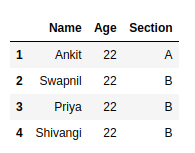Now let’s see different ways of iterate or certain columns of a DataFrame :

Method #1: Using DataFrame.iteritems():
Dataframe class provides a member function `iteritems()` which gives an iterator that can be utilized to iterate over all the columns of a data frame. For every column in the Dataframe it returns an iterator to the tuple containing the column name and its contents as series.

Code :

 `import` `pandas as pd  ` ` `  ` `  `# List of Tuples ` `students ``=` `[(``'Ankit'``, ``22``, ``'A'``), ` `           ``(``'Swapnil'``, ``22``, ``'B'``), ` `           ``(``'Priya'``, ``22``, ``'B'``), ` `           ``(``'Shivangi'``, ``22``, ``'B'``), ` `            ``] ` ` `  `# Create a DataFrame object ` `stu_df ``=` `pd.DataFrame(students, columns ``=``[``'Name'``, ``'Age'``, ``'Section'``],  ` `                      ``index ``=``[``'1'``, ``'2'``, ``'3'``, ``'4'``]) ` ` `  `# gives a tuple of column name and series ` `# for each column in the dataframe ` `for` `(columnName, columnData) ``in` `stu_df.iteritems(): ` `    ``print``(``'Colunm Name : '``, columnName) ` `    ``print``(``'Column Contents : '``, columnData.values) `

Output: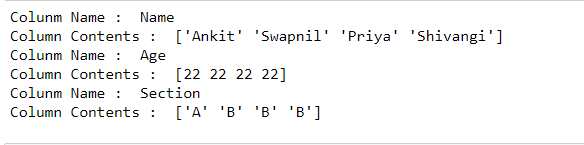Method #2: Using [ ] operator :
We can iterate over column names and select our desired column.

Code :

 `import` `pandas as pd  ` ` `  ` `  `# List of Tuples ` `students ``=` `[(``'Ankit'``, ``22``, ``'A'``), ` `           ``(``'Swapnil'``, ``22``, ``'B'``), ` `           ``(``'Priya'``, ``22``, ``'B'``), ` `           ``(``'Shivangi'``, ``22``, ``'B'``), ` `            ``] ` ` `  `# Create a DataFrame object ` `stu_df ``=` `pd.DataFrame(students, columns ``=``[``'Name'``, ``'Age'``, ``'Section'``], ` `                      ``index ``=``[``'1'``, ``'2'``, ``'3'``, ``'4'``]) ` ` `  `# Iterate over column names ` `for` `column ``in` `stu_df: ` `     `  `    ``# Select column contents by column ` `    ``# name using [] operator ` `    ``columnSeriesObj ``=` `stu_df[column] ` `    ``print``(``'Colunm Name : '``, column) ` `    ``print``(``'Column Contents : '``, columnSeriesObj.values) `

Output: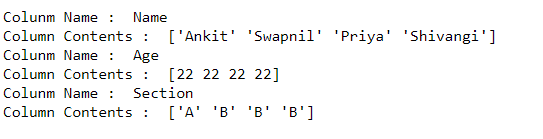Method #3: Iterate over more than one column :
Assume we need to iterate more than one column. In order to do that we can choose more than one column from dataframe and iterate over them.

Code :

 `import` `pandas as pd  ` ` `  ` `  `# List of Tuples ` `students ``=` `[(``'Ankit'``, ``22``, ``'A'``), ` `           ``(``'Swapnil'``, ``22``, ``'B'``), ` `           ``(``'Priya'``, ``22``, ``'B'``), ` `           ``(``'Shivangi'``, ``22``, ``'B'``), ` `            ``] ` ` `  `# Create a DataFrame object ` `stu_df ``=` `pd.DataFrame(students, columns ``=``[``'Name'``, ``'Age'``, ``'Section'``],  ` `                      ``index ``=``[``'1'``, ``'2'``, ``'3'``, ``'4'``]) ` ` `  `# Iterate over two given columns ` `# only from the dataframe ` `for` `column ``in` `stu_df[[``'Name'``, ``'Section'``]]: ` `    `  `    ``# Select column contents by column    ` `    ``# name using [] operator ` `    ``columnSeriesObj ``=` `stu_df[column] ` `    ``print``(``'Colunm Name : '``, column) ` `    ``print``(``'Column Contents : '``, columnSeriesObj.values) `

Output: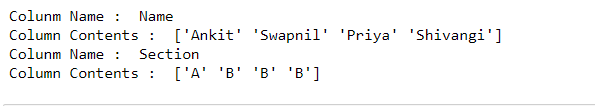Method #4: Iterating columns in reverse order :
We can iterate over columns in reverse order as well.

Code :

 `import` `pandas as pd  ` ` `  ` `  `# List of Tuples ` `students ``=` `[(``'Ankit'``, ``22``, ``'A'``), ` `           ``(``'Swapnil'``, ``22``, ``'B'``), ` `           ``(``'Priya'``, ``22``, ``'B'``), ` `           ``(``'Shivangi'``, ``22``, ``'B'``), ` `            ``] ` ` `  `# Create a DataFrame object ` `stu_df ``=` `pd.DataFrame(students, columns ``=``[``'Name'``, ``'Age'``, ``'Section'``], ` `                       ``index ``=``[``'1'``, ``'2'``, ``'3'``, ``'4'``]) ` ` `  ` `  `# Iterate over the sequence of column names ` `# in reverse order ` `for` `column ``in` `reversed``(stu_df.columns): ` `    `  `    ``# Select column contents by column ` `    ``# name using [] operator ` `    ``columnSeriesObj ``=` `stu_df[column] ` `    ``print``(``'Colunm Name : '``, column) ` `    ``print``(``'Column Contents : '``, columnSeriesObj.values) `

Output: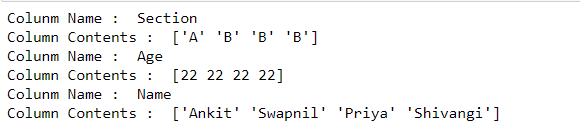Method #5: Using index (iloc) :
To iterate over the columns of a Dataframe by index we can iterate over a range i.e. 0 to Max number of columns than for each index we can select the contents of the column using iloc[].

Code :

 `import` `pandas as pd  ` ` `  ` `  `# List of Tuples ` `students ``=` `[(``'Ankit'``, ``22``, ``'A'``), ` `           ``(``'Swapnil'``, ``22``, ``'B'``), ` `           ``(``'Priya'``, ``22``, ``'B'``), ` `           ``(``'Shivangi'``, ``22``, ``'B'``), ` `            ``] ` ` `  `# Create a DataFrame object ` `stu_df ``=` `pd.DataFrame(students, columns ``=``[``'Name'``, ``'Age'``, ``'Section'``],  ` `                      ``index ``=``[``'1'``, ``'2'``, ``'3'``, ``'4'``]) ` ` `  ` `  `# Iterate over the index range from  ` `# 0 to max number of columns in dataframe ` `for` `index ``in` `range``(stu_df.shape[``1``]): ` `    `  `    ``print``(``'Column Number : '``, index) ` `     `  `    ``# Select column by index position using iloc[] ` `    ``columnSeriesObj ``=` `stu_df.iloc[:, index] ` `    ``print``(``'Column Contents : '``, columnSeriesObj.values) `

Output: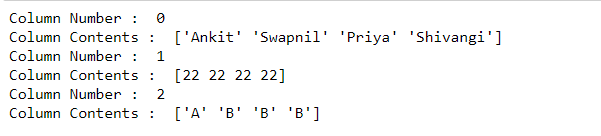My Personal Notes arrow_drop_upCheck out this Author's contributed articles.

If you like GeeksforGeeks and would like to contribute, you can also write an article using contribute.geeksforgeeks.org or mail your article to contribute@geeksforgeeks.org. See your article appearing on the GeeksforGeeks main page and help other Geeks.

Please Improve this article if you find anything incorrect by clicking on the "Improve Article" button below.

Article Tags :

1

Please write to us at contribute@geeksforgeeks.org to report any issue with the above content.